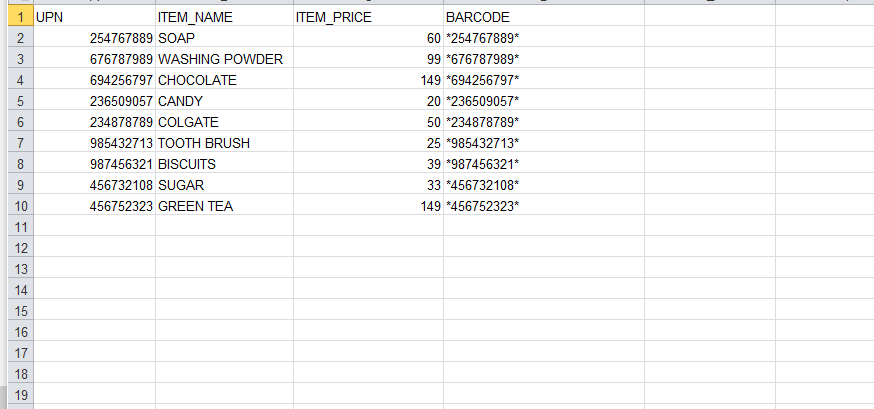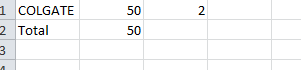Hey folks!! Have you ever been to a grocery store or a shopping mall and hated to stand in a long queue for billing? If yes, then you must have thought of a system using which there’s no need to stand in the long queue. So, my friends, in today’s blog ,we are going to develop a solution where you can simply checkout with your stuff without standing in the long hectic queue.

You must have seen the person on the billing counter scans the barcode of the product you bought. Wonder, if you are able to scan the barcode using your smartphone, then there’s no need to stand in the queue.

Now, you must be wondering, is it possible to make such an application?

The answer to your question is YES. You can develop a barcode detection system using OpenCV.

Import necessary libraries

import cv2
import numpy as np
import pyzbar.pyzbar as pyzbar
import xlrd
import xlsxwriter

To develop barcode detection application, we are importing the following libraries

• CV2 for reading the image
• Numpy
• pyzbar for detecting the barcode and information associated with it
• xlrd for reading the excel file
• xlsxwriter for writing in excel file

After importing necessary libraries, I will give the location of an excel file where I have written the information regarding different products you see in the grocery store.

loc = (r"Grocery Items list.xlsx")
wb = xlrd.open_workbook(loc)
sheet = wb.sheet_by_index(0)
sheet.cell_value(0, 0)Barcode detection

To detect the barcode, I’ll be asking the user to input the quantity bought and barcode to calculate the bill. As of now, I’m using below image as input.

qty=int(input("ENTER THE QUANTITY OF THE PRODUCT:"))

Now, for getting the information associated with barcode, I will use the pyzbar.decode() function which provides us with the unique number of barcode.

decodeObjects = pyzbar.decode(img)
print(decodeObjects)

Now, I will be converting that unique number, which is in binary format, to decimal number so that I can search for it in my workbook. If it is present in the sheet, all information related to that number will be fetched from the file.

for obj in decodeObjects:
b=int(obj.data)
print(b)

Lastly, if the number matches I will be saving the quantity, item name and item price and total in different rows for generating the bill.

for i in range(sheet.nrows):
if sheet.cell_value(i,0)==b:
s=sheet.row_values(i)
worksheet.write(row,col, s)
worksheet.write(row,col+1,s)
worksheet.write(row,col+2,qty)
row+=1
total=total+(s*qty)
worksheet.write(row, 0, 'Total')
worksheet.write(row, 1, total)

workbook.close()

I’ll save the information in a file which will look as follows:Conclusion

So guys, I have implemented the barcode detection program. I hope this helps you. Try to implement it on your own.

-Shruti Sharma

$${}$$